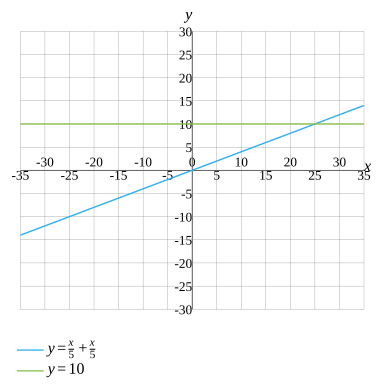\frac{x}{5}+\frac{x}{5}=10
Question
\frac{x}{5}+\frac{x}{5}=10
Solve the equation
\text{Solve for }x
x=25
Evaluate
\frac{x}{5}+\frac{x}{5}=10
Multiply both sides of the equation by LCM
5\left(\frac{x}{5}+\frac{x}{5}\right)=5\times 10
Calculate
5\times \frac{x}{5}+5\times \frac{x}{5}=5\times 10
Calculate
More Steps Hide Steps
Evaluate
5\times \frac{x}{5}+5\times \frac{x}{5}
Reduce the numbers
x+5\times \frac{x}{5}
Reduce the numbers
x+x
Collect like terms
\left(1+1\right)\times x
2x
2x=5\times 10
Multiply the numbers
2x=50
Divide both sides
\frac{2x}{2}=\frac{50}{2}
Simplify
More Steps Hide Steps
Evaluate
\frac{50}{2}
Rewrite the expression
\frac{25\times 2}{2}
Reduce the fraction
25
\frac{2x}{2}=25
Solution
x=25
Rewrite the equation
Rewrite in standard form
x=25
Evaluate
\frac{x}{5}+\frac{x}{5}=10
Evaluate
\frac{2x}{5}=10
Multiply both sides of the equation by LCD
2x=50
Solution
x=25
Graph